11.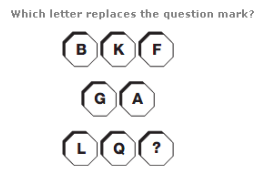A. J B. Y C. R D. V

Explanation: :

Starting at the top left, and moving anti-clockwise around the figure, letters advance through the alphabet 5 letters at a time.

12.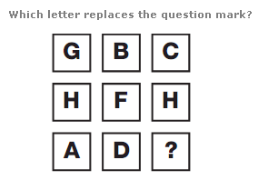A. E B. Z C. X D. C

Explanation: :

Using the numerical value of each letter, in each column, the value of the central letter equals the sum of the values of the top and bottom letters.

13.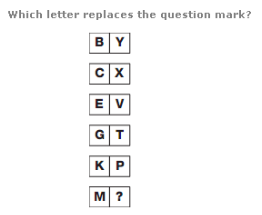A. N B. I C. L D. E

Explanation: :

The alphabetical value of the letters in the left hand column follows through the sequence of Prime Numbers, with the letters in the right hand column representing the corresponding reverse alphabetical values.

14.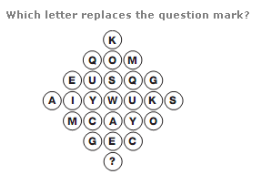A. U B. V C. C D. I

Explanation: :

Starting on the left, and moving downwards in columns from left to right, letters are written in alphabetical sequence, in steps of 4 letters at a time.

15.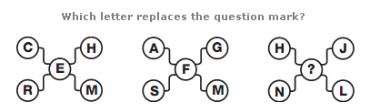A. S B. P C. L D. B